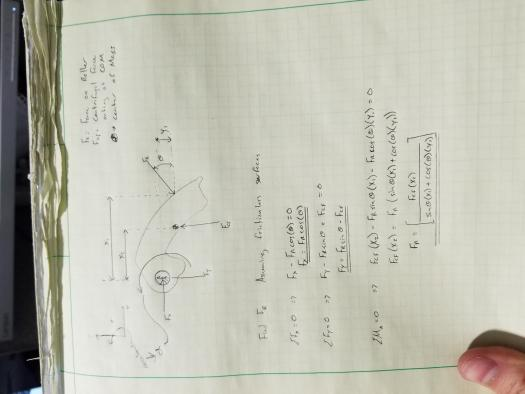# Acceleration of Riged body (flyweight) with forces

1 post / 0 new
kmobile321
Acceleration of Riged body (flyweight) with forces

I have a flyweight that rotates about a pin in a cvt clutch.
the flyweight gets its motion from centrifugal force that acts on the center of gravity on the object. i am trying to develop the acceleration equation using kinamatics but am a little rusty. i have developed the force equations but would like help with the acceleration.• Mathematics inside the configured delimiters is rendered by MathJax. The default math delimiters are $$...$$ and $...$ for displayed mathematics, and $...$ and $...$ for in-line mathematics.